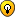## Can you see the next move?

For fans of Kakuro

### Can you see the next move?Jeff

Posts: 708
Joined: 01 August 2005

From the position you posted I would look at 'column' 8. There are only 2 places for a 4.

If the 4 is in row 5 then r5c7=1
If the 4 is in row 6 then r6c7=1, r6c6=5

Either way, 1 can be eliminated from other cells in column 7. This leaves r4c7 as being 2 or 5, so the 8 cage (is that the right term in a kakuro?) in column 7 must be 1,5,2.

Can you progress from that point?
jimbob

Posts: 47
Joined: 07 March 2006

Okay, since r4c7 has to be 2|5, r4c8 cannot be 6.
And if r6c8=6, r6c6=3 => r6c7=1 => r5c7=2 => r5c8=3, and we must have 9s in both r28c8... Therefore r6c8 cannot be 6.
Which leaves r8c8=6, r8c5=1 and r7c5=7, and also r2c8=9...

And the rest would flow from there...udosuk

Posts: 2698
Joined: 17 July 2005

Another way:
Since R7C45 <> {15} -> R7C6 <> 2
-> R6C6 <> 5
Since R6C6 <> {15} -> R6C8 <> 4
-> R5C8 = 4 (hidden single in C8)
R5C7 = 1, R46C7 = , R4C68 = 
...
Jean-Christophe

Posts: 149
Joined: 22 January 2006

Good moves, thank you very much.
Jeff

Posts: 708
Joined: 01 August 2005